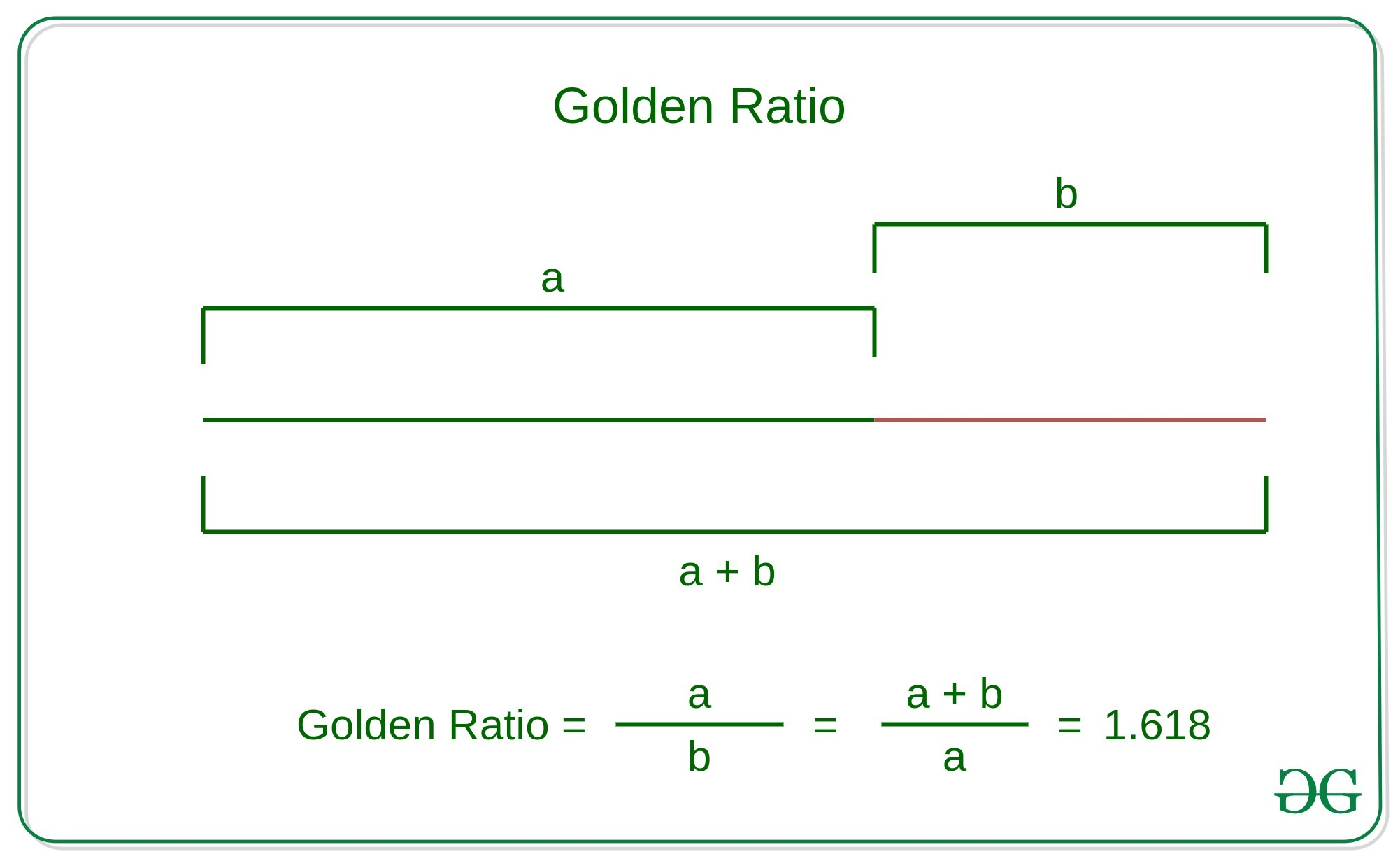# Check whether two numbers are in golden ratio

• Difficulty Level : Expert
• Last Updated : 17 May, 2021

Given two numbers A and B, the task is to check that A and B are in the golden ratio.
Golden Ratio: Two numbers are said to be in the golden ratio if their ratio is the same as the ratio of the sum of the two numbers to the larger number. Here a > b > 0, Below is the geometric representation of the Golden ratio:Attention reader! Don’t stop learning now. Get hold of all the important DSA concepts with the DSA Self Paced Course at a student-friendly price and become industry ready.  To complete your preparation from learning a language to DS Algo and many more,  please refer Complete Interview Preparation Course.

In case you wish to attend live classes with experts, please refer DSA Live Classes for Working Professionals and Competitive Programming Live for Students.Examples:

Input: A = 1, B = 0.618
Output: Yes
Explanation:
These two numbers together forms Golden ratioInput: A = 61.77, B = 38.22

Output Yes

Explanation:

These two numbers together forms Golden ratioApproach: The idea is to find two ratios and check that this ratio is equal to the Golden ratio. That is 1.618.

// Here A denotes the larger numberBelow is the implementation of the above approach:

## C++

 // C++ implementation to check   // whether two numbers are in   // golden ratio with each other  #include  using namespace std;   // Function to check that two   // numbers are in golden ratio  bool checkGoldenRatio(float a,                        float b) {   // Swapping the numbers such     // that A contains the maximum    // number between these numbers    if(a <= b)   {     float temp = a;     a = b;     b = temp;   }     // First Ratio   std::stringstream ratio1;   ratio1 << std :: fixed <<              std :: setprecision(3) <<              (a / b);     // Second Ratio    std::stringstream ratio2;   ratio2 << std :: fixed <<              std :: setprecision(3) <<              (a + b) / a;     // Condition to check that two    // numbers are in golden ratio    if((ratio1.str() == ratio2.str()) &&        ratio1.str() == "1.618")   {     cout << "Yes" << endl;     return true;   }   else   {     cout << "No" << endl;     return false;   } }    // Driver code int main() {   float a = 0.618;   float b = 1;     // Function Call    checkGoldenRatio(a, b);      return 0; }   // This code is contributed by divyeshrabadiya07

## Java

 // Java implementation to check   // whether two numbers are in   // golden ratio with each other  class GFG{       // Function to check that two   // numbers are in golden ratio  public static Boolean checkGoldenRatio(float a,                                        float b) {           // Swapping the numbers such       // that A contains the maximum      // number between these numbers      if (a <= b)     {         float temp = a;         a = b;         b = temp;     }           // First Ratio     String ratio1 = String.format("%.3f", a / b);           // Second Ratio      String ratio2 = String.format("%.3f", (a + b) / a);           // Condition to check that two      // numbers are in golden ratio     if (ratio1.equals(ratio2) &&          ratio1.equals("1.618"))     {         System.out.println("Yes");         return true;     }     else     {         System.out.println("No");            return false;     } }   // Driver code  public static void main(String []args) {     float a = (float)0.618;     float b = 1;           // Function Call      checkGoldenRatio(a, b); } }   // This code is contributed by rag2127

## Python3

 # Python3 implementation to check  # whether two numbers are in  # golden ratio with each other   # Function to check that two  # numbers are in golden ratio def checkGoldenRatio(a, b):           # Swapping the numbers such      # that A contains the maximum     # number between these numbers     a, b = max(a, b), min(a, b)           # First Ratio     ratio1 = round(a/b, 3)           # Second Ratio     ratio2 = round((a+b)/a, 3)     # Condition to check that two     # numbers are in golden ratio     if ratio1 == ratio2 and\        ratio1 == 1.618:         print("Yes")         return True     else:         print("No")         return False           # Driver Code if __name__ == "__main__":     a = 0.618     b = 1           # Function Call     checkGoldenRatio(a, b)

## C#

 // C# implementation to check   // whether two numbers are in   // golden ratio with each other  using System; using System.Collections.Generic; class GFG {           // Function to check that two       // numbers are in golden ratio      static bool checkGoldenRatio(float a,                            float b)     {       // Swapping the numbers such         // that A contains the maximum        // number between these numbers        if(a <= b)       {         float temp = a;         a = b;         b = temp;       }              // First Ratio       string ratio1 = String.Format("{0:0.000}", a / b);              // Second Ratio        string ratio2 = String.Format("{0:0.000}", (a + b) / a);         // Condition to check that two        // numbers are in golden ratio        if(ratio1 == ratio2 && ratio1 == "1.618")       {         Console.WriteLine("Yes");         return true;       }       else       {         Console.WriteLine("No");         return false;       }     }        // Driver code     static void Main() {       float a = (float)0.618;       float b = 1;              // Function Call        checkGoldenRatio(a, b);    } }   // This code is contributed by divyesh072019

## Javascript

 

Output:

Yes

References: https://en.wikipedia.org/wiki/Golden_ratio

My Personal Notes arrow_drop_up
Recommended Articles
Page :